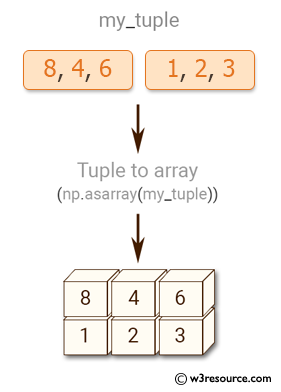﻿ NumPy: Convert a list and tuple into arrays - w3resource# NumPy: Convert a list and tuple into arrays

## NumPy: Array Object Exercise-11 with Solution

Write a NumPy program to convert a list and tuple into arrays.Sample Solution:-

NumPy Code:

``````import numpy as np
my_list = [1, 2, 3, 4, 5, 6, 7, 8]
print("List to array: ")
print(np.asarray(my_list))
my_tuple = ([8, 4, 6], [1, 2, 3])
print("Tuple to array: ")
print(np.asarray(my_tuple))
```
```

Sample Output:

```List to array:
[1 2 3 4 5 6 7 8]
Tuple to array:
[[8 4 6]
[1 2 3]]
```

Python Code Editor:

Have another way to solve this solution? Contribute your code (and comments) through Disqus.

What is the difficulty level of this exercise?

Test your Python skills with w3resource's quiz

﻿

## Python: Tips of the Day

Set comprehension:

```>>> m = {x ** 2 for x in range(5)}
>>> m
{0, 1, 4, 9, 16}
```